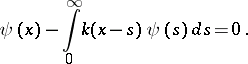# Wiener-Hopf equation

(Redirected from Wiener–Hopf equation)

An integral equation on the half-line with a kernel which depends on the difference between the arguments: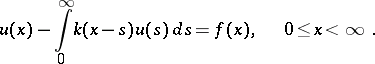(1)

Equations of this type often appear in problems of mathematical physics, e.g. in the theory of radiative transfer (Milne's problem); in the theory of diffraction (diffraction on a half-plane, the problem of boundary refraction).

The first studies of equation (1) are due to N. Wiener and E. Hopf ( and ), and deal with a factorization method (see Wiener–Hopf method). It was the idea of factorization which proved to be the determining factor in the construction of the theory of integral equations such as (1). V.A. Fok (also written as V.A. Fock)  studied the Wiener–Hopf equation on the assumption that the kernelis even and decreases exponentially.

The formal scheme for solving the Wiener–Hopf equation is the following. Let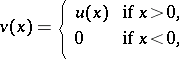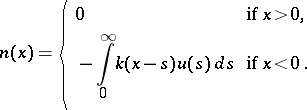Equation (1) can then be written on the whole line as:(2)

If the conditions for the existence of the Fourier transforms of all functions forming part of equation (2), i.e.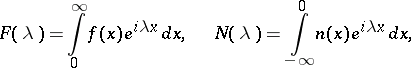are met, then, using the Fourier transform, equation (2) is reduced to the functional equation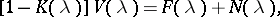(3)

where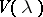and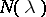are unknown functions. The Wiener–Hopf method makes it possible to solve equation (3) for a certain class of functions. The conditionmust be met in this context. The index of the equation,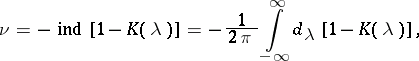(4)

plays a special role in the theory of equation (1) for a non-symmetric kernel. If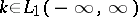and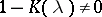, then: if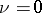, the inhomogeneous equation (1) has a unique solution; if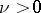, the homogeneous equation (1) haslinearly independent solutions; if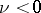, the inhomogeneous equation (1) has either no solution, or else has a unique solution if the following condition is met: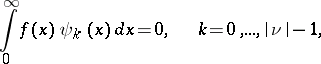where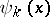are the linearly independent solutions of the transposed homogeneous equation to (1):